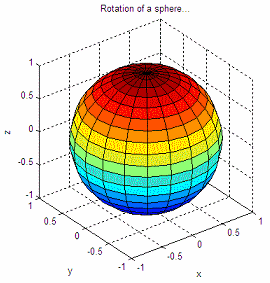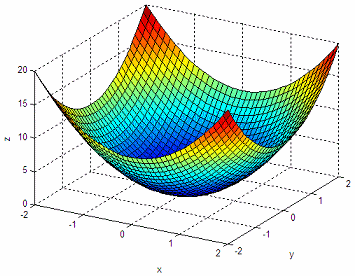3D Simple Animation

 In this short article we’re going to experiment with simple animation in 3D using Matlab. In the first experiment, we are going to work with a sphere and are going to rotate our view angle without changing any size. In the second experiment, we’re going to draw a paraboloid, change its size and rotate. These basic techniques are the foundation of 3D animation with Matlab.

1. Working with a sphere

In this experiment we’re going to draw a sphere and make sure that the axis box keeps its proportions correctly. Then, we’re going to rotate our view angle, i.e., the azimuth and elevation. We are neither moving the sphere itself nor resizing it, we’re just changing our perspective (angle).

Instruction ‘drawnow’ is used to update the current figure. It ‘flushes the event queue’ and forces Matlab to update the screen.

The code that accomplishes this is the following:

clear; clc; close all

% Draw a sphere
sphere
% Make the current axis box square in size
axis('square')

% Define title and labels for reference
title('Rotation of a sphere...')
xlabel(
'x'); ylabel('y'); zlabel('z')

% Modify azimuth (horizontal rotation) and update drawing
for az = -50 : .2 : 30
view(az, 40)
drawnow

end

% Modify elevation (vertical rotation) and update drawing
for el = 40 : -.2 : -30
view(30, el)
drawnow

endand after the two soft rotations we end with an image like this:2. Working with a paraboloid

In our second experiment, we’re going to work with a paraboloid. We first draw it and make sure that the axes have the correct fixed sizes for our purposes. We stretch the figure little-by-little and actually see the change in dimensions. We just update the ‘z’ values within a loop using the function ‘set’ (used to modify the handle graphics properties). Finally, we rotate the azimuth of the figure to achive another view of the box. That’s how we achieve this simple animation in 3D.

The code is the following:

clear; clc; close all

% Define paraboloid
X = -2 : .1 : 2; Y = X;
[x, y] = meshgrid(X, Y);
z = .5 * (x.^2 + y.^2);

% Draw 3D figure, keep track of its handle
h = surf(x,y,z);
% Keep axes constant
axis([-2 2 -2 2 0 20])

% Define title and labels for reference
xlabel('x'); ylabel('y'); zlabel('z')

% Stretch paraboloid and show updates
for i = 1 : .1 : 5;
set(h,
'xdata', x, 'ydata', y, 'zdata', i*z)
drawnow

end

% Modify azimuth (horizontal rotation) and update drawing
for az = -37.5 : .5 : 30
view(az, 30)
drawnow

endAnd we end up with this one:Done!
These are our first experiments with 3D animations.

From 'Simple Animation 3D' to home

From 'Simple Animation 3D' to 3D Menu

 Top 2D animations